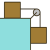Games
Problems
Go Pro!

# Unit 2: Dynamics - Causes of Motion

Reference > Science > Physics > Study GuideNewton's Law of Equilibrium, and Newton's Law of Acceleration - Newton's first two laws of motionUse Newton's Law of Gravity to calculate the gravitational force between two objectsCalculating the amount of friction force between two objects using the coefficient of frictionSolving force and friction problems which involve multiple bodies acting on one another# Blogs on This SiteReviews and book lists - books we love!The site administrator fields questions from visitors.Like us on Facebook to get updates about new resources